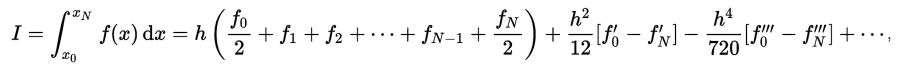## Thursday, June 2, 2016

### ## Integrating on a spherical grid

This post arises from my integration of the NCEP/NCAR reanalysis, described here and here. I have been a little discontented with my method of integrating on a regular lat/lon grid on a sphere. I tried what is usually a very good method - direct trapezoidal integration, with cos weighting for latitude. It worked fairly well, but there was an oddity, in that it gave zero weight to the pole values. That didn't seem right, so I used another method in which cells were weighted by the midpoint values of cos latitude, but with the temperature average used for the cells. That gave finite weighting to the poles, but I still wasn't sure if it was right.

I should emphasise that this is a very minor problem. There are 10226 separate values to integrate, so if two are wrongly weighted, that probably doesn't make a difference at three significant figures. However, I was motivated to review it by some recent posts by Walter Dnes at WUWT. He is doing something similar, and apparently carefully and well. He has cross-checked with my calculations, and reports good agreement with small discrepancies in the third significant figure. Since I found good agreement with NOAA PSD too, that is all reassuring. However, I would like to resolve discrepancies if possible.

There are many possible sources. Calculating the anomaly base is one. I mentioned in this post some leap year issues; that is a very likely source. But I thought I should in any case review my treatment near the poles.

The cos-weighted integral of the temperature T is
∫ T(θ,ψ) cos(θ) dθ d ψ
where θ is latitude, ψ longitude, and the integral is over the sphere. We have values of T on a 2° grid. We can take it that integrating over ψ for each latitude is just 2π times the latitude average (by spherical symmetry). So the question is, how to weight the grid values over θ.

There is an old, interesting and powerful method for generating weights, which is the Euler-MacLaurin formula (wiki). The formula in full generality is given here. I'll show a simpler version:In that form, the f is my T*cos(θ). I'll concentrate on the zero endpoint, being say the S pole. Then the first part of the approximation is the trapezoidal integral, but the pole terms are zero, since cos(π/2)=0. So we must look to the next term. Evaluating f' by the product rule,then f'=T'*cos(-π/2)-T*sin(-π/2)=T. So the extra term changes no other weights, except for adding h²/12 at the poles, and this gives second order accuracy.

So I am now using this scheme, and it makes a very small difference. Daily averages can vary by a small integer in the third significant figures; monthly averages mostly agree exactly, with an occasional discrepancy of 1 in the third figure. So it doesn't explain the somewhat larger discrepancies with Walter's results. However, as I noted here, differences in leap year treatment can have effects of that order.

I haven't yet recalculated earlier years or the anomaly base, but I don't expect that will make any difference.

#### Appendix

There is an interesting way of seeing the Euler-MacLaurin theorem in terms of another old and powerful theory - the difference calculus. The basis of this is that the increment operator
Δf(x)=f(x+h)-f(x)=(exp(hD)-1)f, where D is differentiation.

This compressed notation reflects the Taylor-MacLaurin theorem:
f(x+h)-f(x)=hf'(x)+(h²/2)f''(x)+...

Then the sum of h-spaced points is the inverse of Δ, and 1/D is the integral, and so
h/Δ=h/(exp(hD)-1)=1/D-h/2+(h²/6)D+...

This is just a Taylor series expansion of 1/(exp)x)-1), and the Bernoulli numbers of the general form are just the coefficients of that series.

#### 2 comments:

1.Hello Nick,

I am wanting to contact you. Can you please email me?

I have done some work on analysing trends in eastern Australian soil temperature data.

John Knight
john at johnknight dt com dt australia

1.Hi John,
Emailing now.